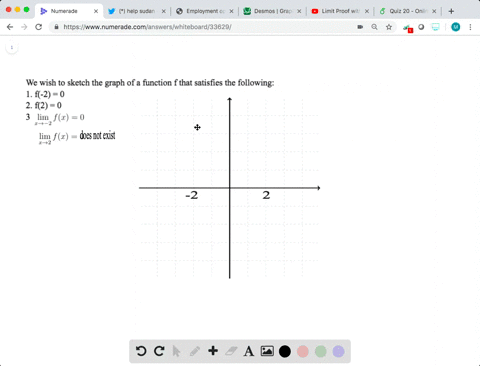Enroll in one of our FREE online STEM bootcamps. Join today and start acing your classes!View Bootcamps### Sketching a Graph In Exercises 33 and $34,$ sketc…

04:30University of California, Berkeley
Problem 33

# Sketching a Graph In Exercises 33 and $34,$ sketch a graph of a function $f$ that satisfies the given values. There are many correct answers.)$f(0)$ is undefined$$\lim _{x \rightarrow 0} f(x)=4$$$$f(2)=6$$$$\lim _{x \rightarrow 2} f(x)=3$$

## Discussion

You must be signed in to discuss.

## Video Transcript

Okay, so we asked us get to graft with you. Fall in, move prime. It's on the left hand side. The freshman is at zero than the fine. But before I do that, that check art. But, ERM Requirement, who's in my, um, be dependent on our requirement. Like, for example, the second restriction, which is a limit as expertise are about the best before this means that every approach, our left and our right hand side of the our function is he good for. So let's see, uh, let's say this is for we should our function and throw it on the fine. So it's your circle over here, and they're now from our left hand side. We can be any function as long as it, uh, touches. All right, approach is, uh, for when exiting. Now, let's see. Ordered bullet to is equal to six. Yeah, and then the limits as XO, just two of our function is equal to drink. So at three, that means our function should be approaching pretty when extra people so we can have something like this and then like that